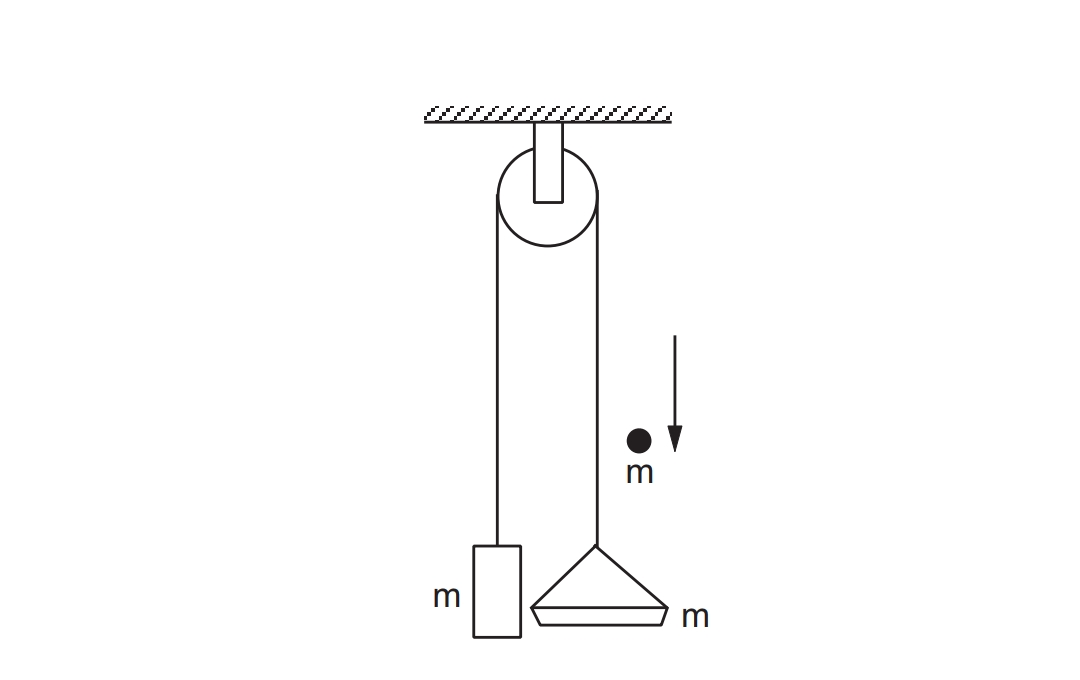# Impulse definition

When a large force acts on a body for very small time, then force is called impulsive force. impulse is defined as the product of force and time.

# Impulse formula

Impulse = force × time = change in momentum.

impulse is a vector quantity and its direction is the direction of the force.

SI unit of impulse is newton second( Ns).

# Impulse and Impulsive force equation and explanation

When two bodies collide, they exert forces on each other while in contact. The momentum of each body is changed due to the force exerted by the other. On an ordinary scale, the time duration of this contact is very small and yet the change in momentum is sizeable. This means that the magnitude of the force must be large on an ordinary scale. Such large forces acting for a very short duration are called impulsive forces.

The force may not be uniform while the contact lasts. The change in momentum produced by such an impulsive force isThis quantity tfti F dt is known as the impulse of the force F during the time interval ti, to tf, and is equal to the change in the momentum of the body on which it acts. It is the area under the F - t curve for one-dimensional motion.

# Impulse important Example

A block of mass m and a pan of equal mass are connected by a string going over a smooth light pulley as shown in the figure. Initially, the system is at rest when a particle of mass m falls on the pan and sticks to it. If the particle strikes the pan with a speed v find the speed with which the system moves just after the collision.

Solution:

Let the required speed be V.

As there is a sudden change in the speed of the block, the tension must change by a large amount during the collision.Let N = magnitude of the contact force between the particle and the pan

T = tension in the string

Thus, Consider the impulse imparted to the particle. The force is N in the upward direction and the impulse is ഽ N dt. This should be equal to the change in its momentum.

thus, ഽN dt = mv – mV ...( i )

Similarly considering the impulse imparted to the pan,

ഽ (N - T ) dt = m V ...( ii )

and that to the block,

ഽ T dt = mV ...( iii )

Adding ( ii ) and ( iii ),

ഽ N dt = 2 m V.

Comparing with ( i ),

mv - mV = 2m V or,

V = v / 3